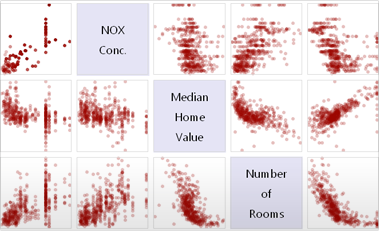# Enhanced Probability & Statistics

Mathematica 9 adds numerous extensions and improvements to its already very strong capabilities in probability and statistics. These include additional parametric distributions, faster nonparametric distributions, additional and generalized derived and formula distributions. A new type of descriptive statistics for measuring dependence among components for multivariate data and distributions has been added, as well as a corresponding set of hypothesis tests. The ability to associate weights with data points allows most data-oriented functions to provide weighted estimators, including descriptive statistics, nonparametric, and parametric distribution estimators. Performance improvements are available across the board.• Weighted data supported throughout nonparametric and parametric estimation.
• Comprehensive collection of multivariate statistical dependency measures. »
• Comprehensive collection of dependency and correlation hypothesis tests.
• Collection of phase-type (hypoexponential, hyperexponential, Coxian) distributions. »
• Additional finance-related parametric distributions (variance-gamma, Tsallis-normal, ...).
• New derived distribution for splicing together multiple distributions, such as body and tail.
• Support for general compound Poisson distributions.
• Order statistics distribution for general multivariate distributions.
• Enhanced performance across the board for nonparametric distributions.
• Support for formula distributions defined by hazard functions.
• Support for random graphs and property distributions of these. »
• Support for Monte Carlo-based probability and expectation computations.

## Mathematica

Questions? Comments? Contact a Wolfram expert »